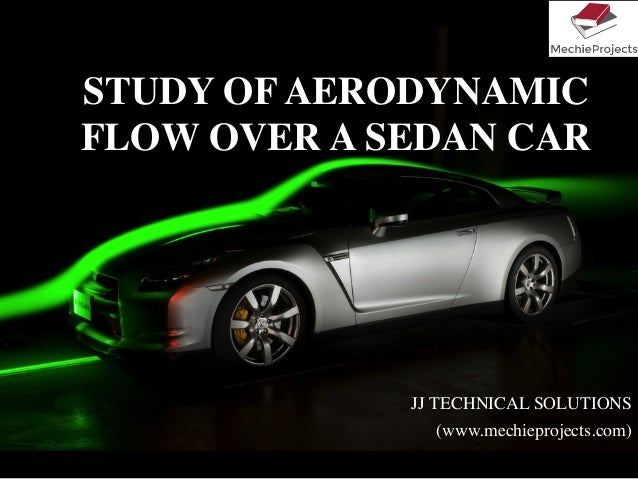Successfully reported this slideshow.Upcoming SlideShare
×

# Aerodynamic Flow over a Car

5,222 views

Published on

The aerodynamic flow over a sedan class car is studied and the various parameters plotted. The coefficient of drag is calculated from the analysis

Published in: Engineering
• Full Name
Comment goes here.

Are you sure you want to Yes No### Aerodynamic Flow over a Car

1. 1. STUDY OF AERODYNAMIC FLOW OVER A SEDAN CAR JJ TECHNICAL SOLUTIONS (www.mechieprojects.com)
2. 2. AIM 1.  To study and understand the aerodynamic air ﬂow over a sedan class car using CFD 2.  To calculate the aero-force acting on the vehicle for different velocities & compute the drag coefﬁcient
3. 3. PROBLEM FORMULATION Tools Used: 1.  Drafting/Modeling: SolidWorks 2.  Meshing: Gambit 3.  Analysis & Post Processing: Fluent Car Velocity Inlet Meshing of Model in Gambit & Deﬁning Boundary Conditions Pressure Outlet Velocity Inlet Wall Velocity of Car Considered 1.  10m/s 2.  15m/s 3.  20m/s 4.  30m/s 5.  40m/s 6.  50m/s 7.  60m/s
4. 4. Problem Deﬁnition in FLUENT Deﬁne  the  problem  as,    Solver      -­‐Pressure  based    Formulation  -­‐Implicit    Space      -­‐2D    Time    -­‐Steady    Viscous    -­‐Two-­‐equation  SST-­‐k-­‐omega  model    Enable  the  Energy  equation    The  ﬂuid  type  used  is  Air  deﬁned  as  ideal  gas    Operating  pressure=  0  Pa
5. 5. LIFT, DRAG, AND MOMENT COEFFICIENTS •  Behavior of L, D, and M depend on α, but also on velocity and altitude •  V∞, ρ ∞, Wing Area (S), Wing Shape, µ ∞, compressibility •  Characterize behavior of L, D, M with coefficients (cl, cd, cm) ( )Re,, 2 1 2 1 3 2 2 ∞ ∞ ∞ ∞ = =≡ = Mfc Scq L ScV M c SccVM m m m α ρ ρ ( )Re,, 2 1 2 1 2 2 2 ∞ ∞ ∞ ∞ = =≡ = Mfc Sq D SV D c ScVD d d d α ρ ρ ( )Re,, 2 1 2 1 1 2 2 ∞ ∞ ∞ ∞ = =≡ = Mfc Sq L SV L c ScVL l l l α ρ ρ Note on Notation: We use lower case, cl, cd, and cm for infinite wings (airfoils) We use upper case, CL, CD, and CM for finite wings
6. 6. PRESSURE COEFFICIENT, CP •  Use non-dimensional description, instead of plotting actual values of pressure •  Pressure distribution in aerodynamic literature often given as Cp •  So why do we care? –  Distribution of Cp leads to value of cl –  Easy to get pressure data in wind tunnels –  Shows effect of M∞ on cl 2 2 1 ∞∞ ∞ ∞ ∞ − = − ≡ V pp q pp Cp ρ
7. 7. Contours of Static Pressure around the Car for Car Velocity =10m/s
8. 8. Contours of Pressure Coeff. around the Car for Car Velocity =10m/s
9. 9. Contours of Total Pressure around the Car for Car Velocity =10m/s
10. 10. Contours of X-dir. Velocity around the Car for Car Velocity =10m/s
11. 11. Contours of Turbulent viscosity around the Car for Car Velocity =10m/s
12. 12. Contours of Velocity magnitude around the Car for Car Velocity =10m/s
13. 13. Pressure coeff. Plot on top & bottom of the Car for Car Velocity =10m/s
14. 14. Contours of X-dir. Velocity at the front of the Car for Car Velocity =10m/s
15. 15. Contours of X-dir. Velocity at the front of the Car for Car Velocity =10m/s
16. 16. Contours of X-dir. Velocity at the rear of the Car for Car Velocity =10m/s
17. 17. Contours of Stream Function around the Car for Car Velocity =10m/s
18. 18. Velocity Vectors around the Car for Car Velocity =10m/s
19. 19. Contours of X. dir. Velocity around the Car for Car Velocity =60m/s
20. 20. Contours of Turbulent Intensity around the Car for Car Velocity =60m/s
21. 21. Vehicle Velocity (m/s) Pressure Force(N) Viscous Force(N) Total Force (N) Pressure Coeff. Viscous Coeff. Total Coeff. Cl Cd 10 34.071352 4.4314715 38.502823 0.2317779 0.0301460 0.26192396 -1.48 0.262 15 74.373342 9.298441 83.671783 0.2248627 0.0281131 0.2529759 -1.64 0.253 20 130.07131 15.765496 145.83681 0.2212097 0.0268120 0.24802177 -1.75 0.248 30 288.49465 33.227231 321.72188 0.21806095 0.0251150 0.24317601 -1.92 0.243 40 508.64031 56.428935 565.06924 0.21625863 0.0239918 0.24025052 -2.03 0.240 50 790.09973 85.12556 875.22529 0.21499312 0.0231634 0.23815653 -2.10 0.238 60 1137.7129 119.11565 1256.8286 0.21498731 0.0225086 0.23749594 -2.18 0.238 RESULTS
22. 22. CONCLUSION 1.  The contours of Velocity, Pressure & Turbulence is plotted, around the sedan car and studied for car velocity changing from 10 - 60m/s. 2.  The velocity increases neat the hood of the car and the pressure decreases 3.  A swirl/ backﬂow is generated at the rear end of the car with negative velocity. 4.  The pressure coefﬁcient is plotted for the top & bottom side of the car. 5.  The pressure force, viscous force and total force acting on the car for different velocities is plotted against car velocity. 6.  The viscous force is a small fraction of total force acting on the car. 7.  The Lift coefﬁcient decreases with the increase in car velocity, 8.  The Drag coefﬁcient (Cd) for the car is = 0.24
23. 23. FOR COMPLETE PRESENTATION, MORE PROJECTS PRESENTATIONS AND PROJECT REPORTS VISIT WWW.MECHIEPROJECTS.COM Email: contactus@mechieprojects.com THANKYOU